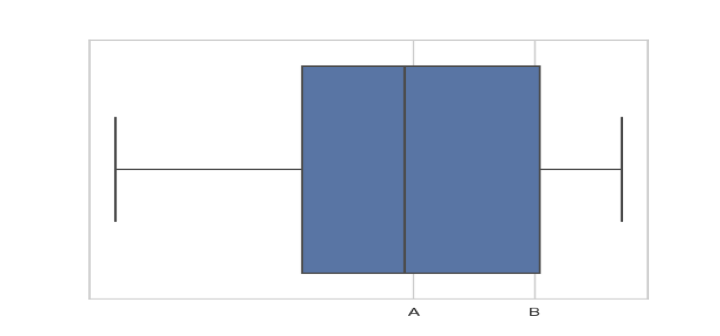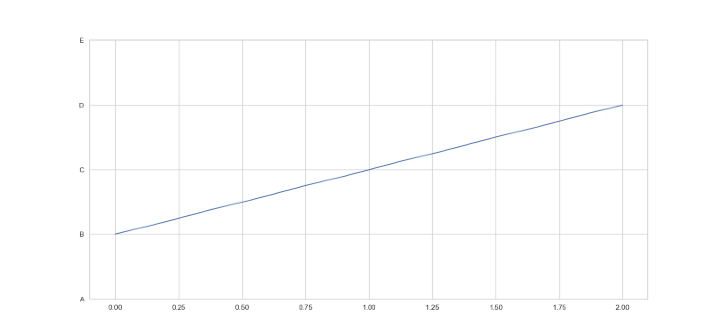# How to Adjust the Number of Ticks in Seaborn Plots?

## Introduction

Ticks are tiny symbols that Matplotlib uses to represent the positions of data points on both axes of a plot. They may be positioned to best fit the data range and are used to highlight certain locations on the x and y axes. Usually, ticks may be labeled to indicate the precise values they stand for. In the python package Seaborn, there are two functions, namely, xticks() and yticks() that can be used for adjusting the ticks of a given graph.

## Syntax

To adjust the number of ticks in Seaborn plots, we can use the following syntax −

# Set the tick locations and labels for the x-axis
ax.set_xticks([tick1, tick2, ...])
ax.set_xticklabels([label1, label2, ...])

# Set the tick locations and labels for the y-axis
ax.set_yticks([tick1, tick2, ...])
ax.set_yticklabels([label1, label2, ...])


Both methods also have an optional minor parameter to set major or minor ticks. Here, ax is the axis object returned by the Seaborn plot function, and tick1, tick2, ... are the desired tick locations, and label1, label2, ... are the corresponding tick labels.

## Algorithm

The general step-by-step algorithm to adjust the number of ticks in Seaborn plots is as follows −

• Choose the Seaborn plotting function you want to use such as sns.scatterplot().

• The sns.set() and sns.set style() routines can be used to change the Seaborn theme and style.

• To plot the data, utilize the chosen Seaborn plotting function.

• Make a variable that points to the plot's axes object.

• To set the number of ticks on the x and/or y axes, use the set xticks() and/or set yticks() methods. A list of tick locations is the parameter for these functions.

• To set the labels for the ticks on the x and/or y axes, use the set xticklabels() and/or set yticklabels() methods. A parameter for these functions is a list of tick labels.

• Plot it on the window with show() method.

## Example

Follow along the example below to make your own Seaborn boxplot with custom tick locations and labels on the x-axis.

import seaborn as sns
import matplotlib.pyplot as plt
import numpy as np

# Generate some random data
data = np.random.randn(20)

# Set up the Seaborn plot
sns.set()
sns.set_style("whitegrid")
ax = sns.boxplot(x=data)

# Set the tick locations and labels, can also use np array here
ax.set_xticks([0, 1])
ax.set_xticklabels(["A", "B"])

# Show the plot
plt.show()

• Using the random.randn function in NumPy, we first create some random data. The set and set style functions are then used to set the visual style for the Seaborn plot.

• By using the boxplot function on the data and saving the generated axis object in the variable axe, we can build a boxplot. The set xticks and set xticklabels methods of the axis object axe are then used to set the tick locations and labels for the x-axis.

• In this instance, we are designating the tick locations as "A" and "B" and setting them to be at positions 0 and 1, respectively. Lastly, we use the pyplot module of matplotlib's show function to display the plot. Be aware that the final plot may not seem particularly fascinating if you execute this code.

• Due to the fact that we are just charting 20 randomly selected data points with just two ticks on the x-axis, the plot that is produced if you execute this code might not appear that fascinating. To produce more illuminating graphs, you may change the code to utilize your own data and adjust the tick placements and labels.Example
import seaborn as sns
import matplotlib.pyplot as plt
import numpy as np

# Generate some random data
data = np.random.randn(20)

# Set up the Seaborn line plot
sns.set()
sns.set_style("whitegrid")
ax = sns.lineplot(x=[0, 1, 2], y=[1, 2, 3])

# Set the ytick locations and labels, can also use np array here
ax.set_yticks([0, 1, 2, 3, 4])
ax.set_yticklabels(["A", "B", "C", "D", "E"])

# Show the plot
plt.show()

• Here, we are generating a line plot using the Seaborn library in Python. The plot has 5 y-ticks with labels "A", "B", "C", "D", and "E".

• Firstly, the Seaborn library is imported along with the Matplotlib library. Then, a NumPy array of random data is generated using the np.random.randn() method.

• Next, the plot is set up using Seaborn with a whitegrid style. The line plot is generated using the sns.lineplot() method with the x-values and y-values specified.

• To adjust the y-ticks, the ax.set_yticks() method is called with a list of values for the y-tick locations. The ax.set_yticklabels() method is then called with a list of labels for the y-ticks.

• Finally, the plot is shown using the plt.show() method.## Conclusion

In this article, we explored and learned that adjusting the number of ticks in Seaborn plots can make our visualizations more informative and easier to read. With the set_xticks() and set_yticks() functions, we can easily adjust the number of ticks and their labels on the x and y axes. We can also use other Seaborn functions to further customize the ticks in our plots. We also saw two hands-on examples which helped us in adjusting the number of x-ticks and y-ticks in our graphs.